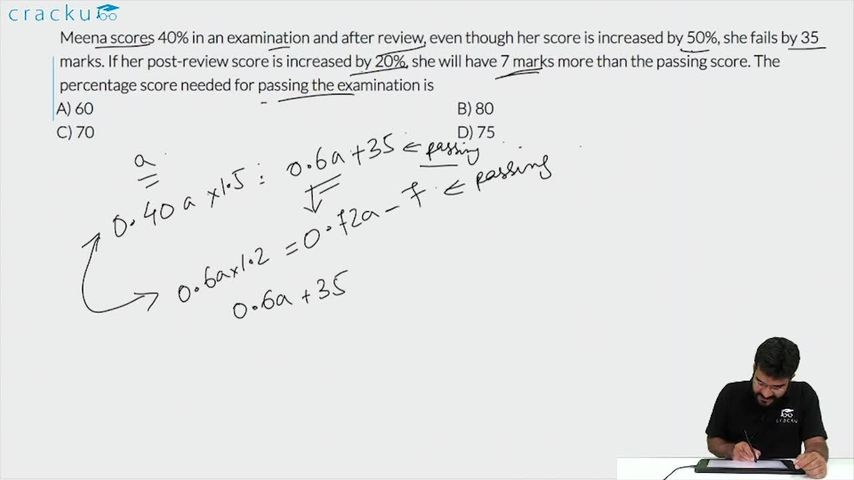Question 81

# Meena scores 40% in an examination and after review, even though her score is increased by 50%, she fails by 35 marks. If her post-review score is increased by 20%, she will have 7 marks more than the passing score. The percentage score needed for passing the examination is

Solution

Assuming the maximum marks =100a, then Meena got 40a

After increasing her score by 50%, she will get 40a(1+50/100)=60a

Passing score = 60a+35

Post review score after 20% increase = 60a*1.2=72a

=>Hence, 60a+35+7=72a

=>12a=42   =>a=3.5

=> maximum marks = 350 and passing marks = 210+35=245

=> Passing percentage = 245*100/350 = 70

### View Video Solution